Dedicated to those who never had the opportunity to receive a good education
Dedicated to those who never had the opportunity to receive a good education
0%

# Force and acceleration in special relativity

Acceleration in special relativity is not parallel to the applied force. This is in contrast to the case of classical mechanics where Newton’s second law implies that force and acceleration are parallel by definition. In the present article, this result is derived in a simple form.

## Force in special relativity

Recall that in special relativity the linear momentum is given by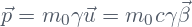. The force is defined as the time derivative of the linear momentum:

(1)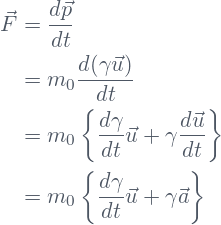The derivative of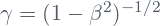, where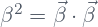, is

(2)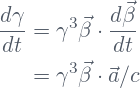Therefore, the expression for the force is

(3)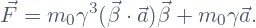## Acceleration is not parallel to the force

Let’s calculate the components of force and acceleration in the directions parallel and perpendicular to the velocity. Let’s start with the parallel components. For the acceleration we have

(4)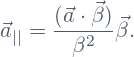In a similar way, the component of the force parallel to the velocity is

(5)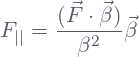If on both sides of Eq.(3) we take the dot product with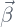,  we obtain the following:

(6)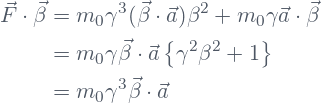To arrive to the last line in Eq.(6), we made use of the identity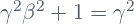, which can be easily verified.

Substituting the result of Eq.(6) in Eq.(5), and using the expression given in Eq.(4),  the following expression for the component of the force parallel to the velocity is obtained:

(7)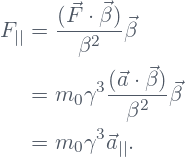The force that acts on the particle can be decomposed in the sum of its components parallel and perpendicular to the direction of the vector velocity . The line in purple corresponds to the trajectory of the particle.

Now let’s calculate the components of force and acceleration perpendicular to the velocity. For the acceleration we have

(8)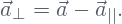For the force, let us note first that with help of Eq.(4), Eq.(7), and Eq.(8), one can rewrite Eq.(3) in the form

(9)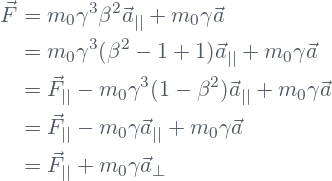Therefore, the component of the force perpendicular to the velocity is

(10)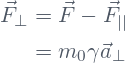From Eq.(7) and Eq.(10) follows that if the applied force is either parallel or perpendicular to the velocity, then the acceleration will be in the same direction of the force. However, in any other case, this is no longer true. To see this, let’s calculate the angles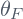, and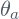that the vectors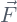and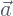form with the velocity vector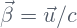. These angles are determined by the following equations:

(11)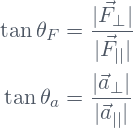The acceleration of the particle is not parallel to the direction of the applied force . The angle between the acceleration and the velocity is related to the angle between the force and the velocity through the relation .

From the definition of these angles, and equations Eq(7), and Eq.(10), the following relation is obtained:

(12)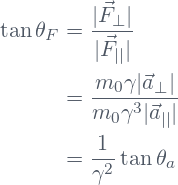From the preceding equation, we obtain the relation between the anglesandthat the force and the acceleration form with respect to the direction of the velocity:

(13)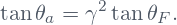This latter relation implies that the acceleration forms an angle with respect to the direction of the velocity that is different from that of the applied force. This in contrast with what happens in classical physics, where by definition, the acceleration is parallel to the applied force.

Share it!